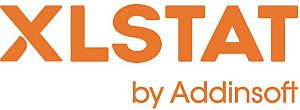# XLSTAT - k-means Clustering

## Principle of k-means Clustering

k-means clustering is an iterative aggregation method which, wherever it starts from, converges on a solution. The solution obtained is not necessarily the same for all starting points. For this reason, the calculations are generally repeated several times in order to choose the optimal solution for the selected criterion.

For the first iteration, a starting point is chosen which consists in associating the center of the k classes with k objects (either taken at random or not).

Afterwards the distance between the objects and the k centers is calculated and the objects are assigned to the centers they are nearest to. Then the centers are redefined from the objects assigned to the various classes. The objects are then reassigned depending on their distances from the new centers. And so on until convergence is reached.

## Use of k-means Clustering

The k-means method is used to divide the observations into homogeneous clusters, based on their description by a set of quantitative variables. k-means clustering has the following advantages in particular:

1. An object may be assigned to a class during one iteration then change class in the following iteration, which is not possible with Agglomerative Hierarchical Clustering for which assignment is irreversible.
2. By multiplying the starting points and the repetitions, several solutions may be explored.

## Classification criteria for k-means Clustering

Several classification criteria may be used to reach a solution. XLSTAT offers four criteria to be minimized:

• Trace(W)
• Determinant(W)
• Wilks lambda
• Trace(W) / Median

## Results for in XLSTAT

• Optimization summary:
This table shows the evolution of the within-class variance. If several repetitions have been requested, the results for each repetition are displayed.
• Statistics for each iteration:
Activate this option to see the evolution of miscellaneous statistics calculated as the iterations for the repetition proceed, given the optimum result for the chosen criterion. If the corresponding option is activated in the Charts tab, a chart showing the evolution of the chosen criterion as the iterations proceed is displayed.
Note: if the values are standardized (option in the Options tab), the results for the optimization summary and the statistics for each iteration are calculated in the standardized space. On the other hand, the following results are displayed in the original space if the "Results in the original space" option is activated.
• Variance decomposition for the optimal classification:
This table shows the within-class variance, the inter-class variance and the total variance.
• Class centroids:
This table shows the class centroids for the various descriptors.
• Distance between the class centroids:
This table shows the Euclidean distances between the class centroids for the various descriptors.
• Central objects:
This table shows the coordinates of the nearest object to the centroid for each class.
• Distance between the central objects:
This table shows the Euclidean distances between the class central objects for the various descriptors.
• Results by class:
The descriptive statistics for the classes (number of objects, sum of weights, within-class variance, minimum distance to the centroid, maximum distance to the centroid, mean distance to the centroid) are displayed in the first part of the table. The second part shows the objects.
• Results by object:
This table shows the assignment class for each object in the initial object order.This analysis is available in the XLStat-Basic addin for Microsoft ExcelKovach Computing Services (KCS) was founded in 1993 by Dr. Warren Kovach. The company specializes in the development and marketing of inexpensive and easy-to-use statistical software for scientists, as well as in data analysis consulting.

### Get in Touch

• Email:
sales@kovcomp.com